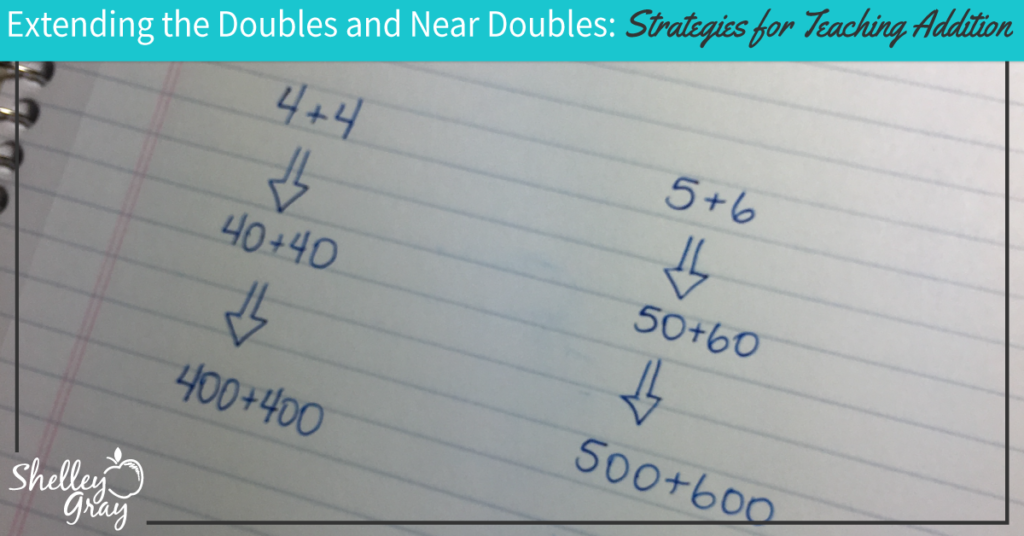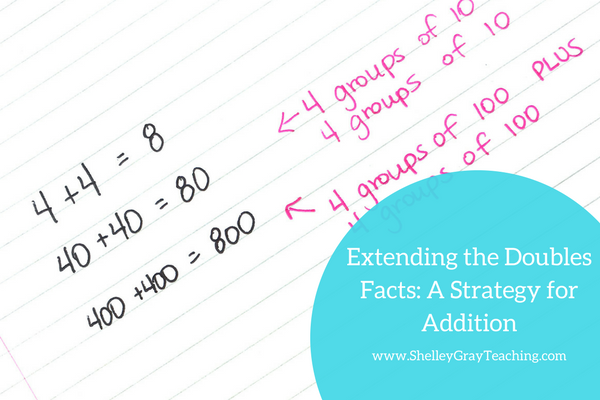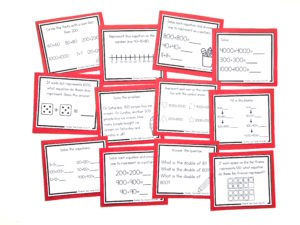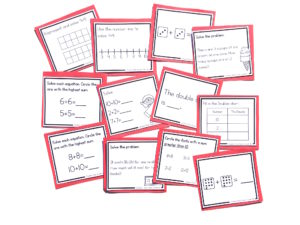# Extending The Doubles and Near Doubles Facts: An Addition StrategyThe doubles facts are generally an introductory set of facts that we want our students to memorize. We can relate the doubles to so many things around us –  fingers and toes: 5+5, wheels on a car : 2+2, or the eggs in a carton: 6+6.

Our goal for the doubles facts is automaticity. This means that students no longer have to think much about the equation in order to solve it. Rather, they just “know” the answer and are able to say the answer within 1-3 seconds. For example, when a student sees the equation 8+8, he should know that it equals 16 without even stopping to think about it.

Building a strong foundation of doubles will help students with other mental math strategies, particularly the near doubles.

Near doubles involve facts like 4+5. To solve this fact before memorization has taken place, we want our students to think, “I know that 4+4=8, and 1 more is 9.”

Teaching the doubles and near doubles facts is important, but it shouldn’t stop simply with the numbers to 12. We need to extend these facts into tens and hundreds as well, and teach our students how we can still use the doubles and near doubles facts in order to solve equations with these bigger numbers.

For example we can use the fact 3+3 to solve 30+30 or the fact 6+6 to solve 60+60=___.

FOCUS ON GROUPS OF 10

To begin teaching students to extend the doubles and near doubles facts, focus on groups of 10. For example, we can think of 40+40 as 4 groups of 10 plus 4 groups of 10. Similarly, to solve 400+400 we can think, “4 groups of 100 plus 4 groups of 100.”EXTENDING THIS CONCEPT TO OTHER MATH STRATEGIES

This “extending” concept can be used in many other circumstances. For example, when teaching your students the plus 1 facts, teach them to extend that knowledge past simple 1-digit numbers. For example, if we know 8+1, then we can easily figure out 80+10 or 800+100.

NEXT STEPS:

• If you would like full support for teaching addition strategies in your classroom, check out The Addition Station HERE.
• Download a FREE activity sheet for practicing the extending the doubles facts strategy HERE.• Find task cards to reinforce the extending the doubles strategy in isolation here: